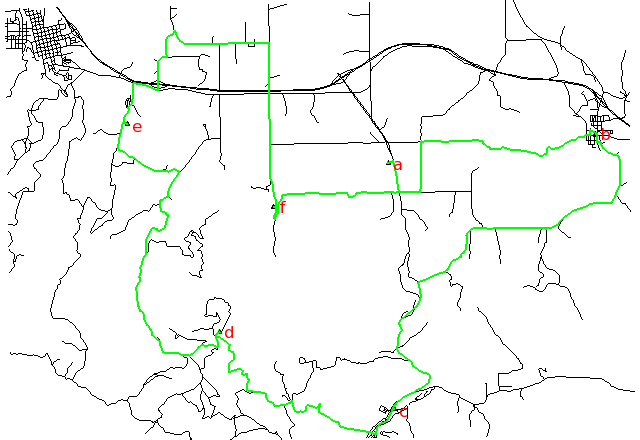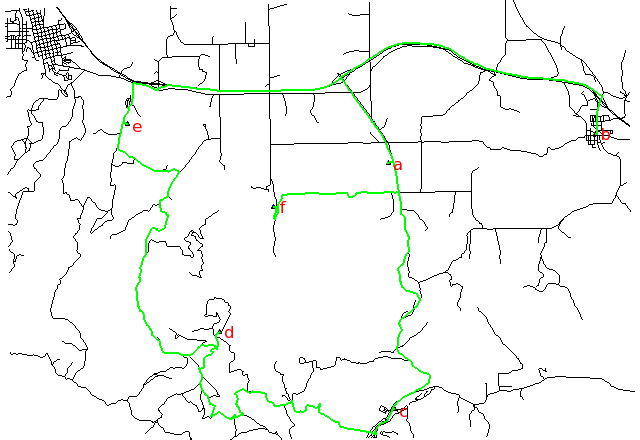Note: A new GRASS GIS stable version has been released: GRASS GIS 7. Go directly to the new manual page here

## NAME

v.net.salesman - Creates a cycle connecting given nodes (Traveling salesman problem).
Note that TSP is NP-hard, heuristic algorithm is used by this module and created cycle may be sub optimal

## KEYWORDS

vector, network, salesman

## SYNOPSIS

v.net.salesman
v.net.salesman help
v.net.salesman [-g] input=name output=name [type=string[,string,...]] [alayer=integer] [nlayer=integer] [acolumn=string] ccats=range [--overwrite] [--verbose] [--quiet]

### Flags:

-g
Use geodesic calculation for longitude-latitude locations
--overwrite
Allow output files to overwrite existing files
--verbose
Verbose module output
--quiet
Quiet module output

### Parameters:

input=name
Name of input vector map
output=name
Name for output vector map
type=string[,string,...]
Arc type
Options: line,boundary
Default: line,boundary
alayer=integer
Layer number
Arc layer
Default: 1
nlayer=integer
Layer number
Node layer (used for cities)
Default: 2
acolumn=string
Arcs' cost column (for both directions)
ccats=range
Category values
Categories of points ('cities') on nodes (layer is specified by nlayer)

## DESCRIPTION

v.net.salesman calculates the optimal route to visit nodes on a vector network.

Costs may be either line lengths, or attributes saved in a database table. These attribute values are taken as costs of whole segments, not as costs to traverse a length unit (e.g. meter) of the segment. For example, if the speed limit is 100 km / h, the cost to traverse a 10 km long road segment must be calculated as
length / speed = 10 km / (100 km/h) = 0.1 h.
Supported are cost assignments for arcs, and also different costs for both directions of a vector line. For areas, costs will be calculated along boundary lines.

The input vector needs to be prepared with v.net operation=connect in order to connect points representing center nodes to the network.

Points specified by category must be exactly on network nodes, and the input vector map needs to be prepared with v.net operation=connect.

## NOTES

Arcs can be closed using cost = -1.

## EXAMPLE

Traveling salesman for 6 digitized nodes (Spearfish):

Shortest path, along unimproved roads:Fastest path, along highways:Searching for the shortest path using distance and the fastest path using traveling time according to the speed limits of different road types:

```# Spearfish

# we have 6 locations to visit on our trip
echo "1|601653.5|4922869.2|a
2|608284|4923776.6|b
3|601845|4914981.9|c
4|596270|4917456.3|d
5|593330.8|4924096.6|e
6|598005.5|4921439.2|f" | v.in.ascii in=- cat=1 x=2 y=3 out=centers col="cat integer, \
east double precision, north double precision, label varchar(43)"

# verify data preparation
v.db.select centers
v.category centers op=report
# type       count        min        max
# point          6          1          6

# create lines map connecting points to network (on layer 2)
# Layer / table: 1 / myroads_net
# type       count        min        max
# line         837          1          5
#
# Layer: 2
# type       count        min        max
# point          6          1          5

# find the shortest path
v.net.salesman myroads_net ccats=1-6 out=mysalesman_distance

# set up costs as traveling time

# create unique categories for each road in layer 3

# add new table for layer 3
v.db.addtable myroads_net_time layer=3 col="cat integer,label varchar(43),length double precision,speed double precision,cost double precision"

# copy road type to layer 3
v.to.db myroads_net_time layer=3 qlayer=1 opt=query qcolumn=label columns=label

v.to.db myroads_net_time layer=3 type=line option=length col=length unit=miles

# set speed limits in miles / hour
v.db.update myroads_net_time layer=3 col=speed val="5.0"
v.db.update myroads_net_time layer=3 col=speed val="75.0" where="label='interstate'"
v.db.update myroads_net_time layer=3 col=speed val="75.0" where="label='primary highway, hard surface'"
v.db.update myroads_net_time layer=3 col=speed val="50.0" where="label='secondary highway, hard surface'"
v.db.update myroads_net_time layer=3 col=speed val="25.0" where="label='light-duty road, improved surface'"
v.db.update myroads_net_time layer=3 col=speed val="5.0" where="label='unimproved road'"

# define traveling costs as traveling time in minutes:
v.db.update myroads_net_time layer=3 col=cost val="length / speed * 60"

# find the fastest path
v.net.salesman myroads_net_time alayer=3 nlayer=2 acol=cost ccats=1-6 out=mysalesman_time
```
To display the result, run for example:
```# Display the results

# shortest path
d.mon x0
d.vect centers -c icon=basic/triangle
d.vect mysalesman_distance col=green width=2
d.font Vera
d.vect centers col=red disp=attr attrcol=label lsize=12

# fastest path
d.mon x1
d.vect centers -c icon=basic/triangle
d.vect mysalesman_time col=green width=2
d.font Vera
d.vect centers col=red disp=attr attrcol=label lsize=12
```